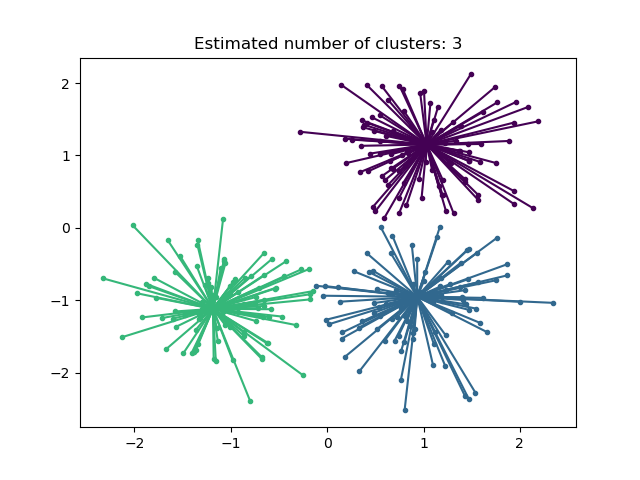# Demo of affinity propagation clustering algorithm¶

Reference: Brendan J. Frey and Delbert Dueck, “Clustering by Passing Messages Between Data Points”, Science Feb. 2007

from sklearn.cluster import AffinityPropagation
from sklearn import metrics
from sklearn.datasets import make_blobs


## Generate sample data¶

centers = [[1, 1], [-1, -1], [1, -1]]
X, labels_true = make_blobs(
n_samples=300, centers=centers, cluster_std=0.5, random_state=0
)


## Compute Affinity Propagation¶

af = AffinityPropagation(preference=-50, random_state=0).fit(X)
cluster_centers_indices = af.cluster_centers_indices_
labels = af.labels_

n_clusters_ = len(cluster_centers_indices)

print("Estimated number of clusters: %d" % n_clusters_)
print("Homogeneity: %0.3f" % metrics.homogeneity_score(labels_true, labels))
print("Completeness: %0.3f" % metrics.completeness_score(labels_true, labels))
print("V-measure: %0.3f" % metrics.v_measure_score(labels_true, labels))
print(
)
print(
"Silhouette Coefficient: %0.3f"
% metrics.silhouette_score(X, labels, metric="sqeuclidean")
)


Out:

Estimated number of clusters: 3
Homogeneity: 0.872
Completeness: 0.872
V-measure: 0.872
Silhouette Coefficient: 0.753


## Plot result¶

import matplotlib.pyplot as plt
from itertools import cycle

plt.close("all")
plt.figure(1)
plt.clf()

colors = cycle("bgrcmykbgrcmykbgrcmykbgrcmyk")
for k, col in zip(range(n_clusters_), colors):
class_members = labels == k
cluster_center = X[cluster_centers_indices[k]]
plt.plot(X[class_members, 0], X[class_members, 1], col + ".")
plt.plot(
cluster_center,
cluster_center,
"o",
markerfacecolor=col,
markeredgecolor="k",
markersize=14,
)
for x in X[class_members]:
plt.plot([cluster_center, x], [cluster_center, x], col)

plt.title("Estimated number of clusters: %d" % n_clusters_)
plt.show()Total running time of the script: ( 0 minutes 0.275 seconds)

Gallery generated by Sphinx-Gallery